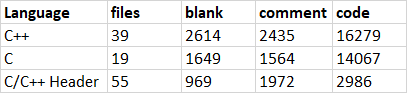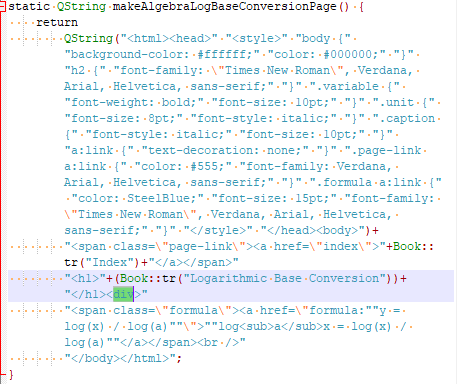﻿ Following in the Footsteps of Calculators: SpeedCrunch# Following in the Footsteps of Calculators: SpeedCrunch

Here we are, continuing to explore the code of calculators! Today we are going to take a look at the project called SpeedCrunch, the second most popular free calculator.## Introduction

SpeedCrunch is a high-precision scientific calculator featuring a fast, keyboard-driven user interface. It is free and open-source software, licensed under the GPL and running on Windows, Linux, and macOS.

The source code is available on BitBucket. I was somewhat disappointed by the build documentation, which could be more detailed. It says that you need "Qt 5.2 or later" to build the project, but it actually required a few specific packages, which wasn't easy to figure out from the CMake log. By the way, it is considered a good practice nowadays to include a Dockerfile into the project to make it easier for the user to set up the development environment.

Here's output from the Cloc utility showing how SpeedCrunch compares to other calculators:Bug reviews for the other projects:

The analysis was done with the PVS-Studio static analyzer. This is a package of solutions for software quality control and detection of bugs and potential vulnerabilities. PVS-Studio supports C, C++, C#, and Java and runs on Windows, Linux, and macOS.

## Strange logic in a loop

V560 A part of conditional expression is always true: !ruleFound. evaluator.cpp 1410

``````void Evaluator::compile(const Tokens& tokens)
{
....
while (!syntaxStack.hasError()) {
bool ruleFound = false;                                     // <=

// Rule for function last argument: id (arg) -> arg.
if (!ruleFound && syntaxStack.itemCount() >= 4) {           // <=
Token par2 = syntaxStack.top();
Token arg = syntaxStack.top(1);
Token par1 = syntaxStack.top(2);
Token id = syntaxStack.top(3);
if (par2.asOperator() == Token::AssociationEnd
&& arg.isOperand()
&& par1.asOperator() == Token::AssociationStart
&& id.isIdentifier())
{
ruleFound = true;                                   // <=
syntaxStack.reduce(4, MAX_PRECEDENCE);
m_codes.append(Opcode(Opcode::Function, argCount));
#ifdef EVALUATOR_DEBUG
dbg << "\tRule for function last argument "
<< argCount << " \n";
#endif
argCount = argStack.empty() ? 0 : argStack.pop();
}
}
....
}
....
}``````

Note the ruleFound variable: it is set to false at each iteration. Inside the loop's body, though, that variable is set to true on certain conditions, but it will be set back to false at the next iteration. The ruleFound variable should have been probably declared before the loop.

## Suspicious comparisons

V560 A part of conditional expression is always true: m_scrollDirection != 0. resultdisplay.cpp 242

``````void ResultDisplay::fullContentScrollEvent()
{
QScrollBar* bar = verticalScrollBar();
int value = bar->value();
bool shouldStop = (m_scrollDirection == -1 && value <= 0) ||
(m_scrollDirection == 1 && value >= bar->maximum());

if (shouldStop && m_scrollDirection != 0) {     // <=
stopActiveScrollingAnimation();
return;
}

scrollLines(m_scrollDirection * 10);
}``````

If the shouldStop variable's value is true, then the m_scrollDirection variable will take one of the two values: -1 or 1. Therefore, its value will definitely be different from zero in the next conditional statement, which is what the analyzer is warning about.

V668 There is no sense in testing the 'item' pointer against null, as the memory was allocated using the 'new' operator. The exception will be generated in the case of memory allocation error. editor.cpp 998

``````void EditorCompletion::showCompletion(const QStringList& choices)
{
....
for (int i = 0; i < choices.count(); ++i) {
QStringList pair = choices.at(i).split(':');
QTreeWidgetItem* item = new QTreeWidgetItem(m_popup, pair);

if (item && m_editor->layoutDirection() == Qt::RightToLeft)
item->setTextAlignment(0, Qt::AlignRight);
....
}
....
}``````

The memory for an object of type QTreeWidgetItem is allocated using the new operator. It means that a memory allocation failure will lead to throwing an std::bad_alloc() exception. Checking the item pointer is, therefore, redundant and can be removed.

## Potential NULL Dereference

V595 The 'ioparams' pointer was utilized before it was verified against nullptr. Check lines: 969, 983. floatio.c 969

``````int cattokens(....)
{
....
if (printexp)
{
if (expbase < 2)
expbase = ioparams->expbase;  // <=
....
}
dot = '.';
expbegin = "(";
expend = ")";
if (ioparams != NULL)            // <=
{
dot = ioparams->dot;
expbegin = ioparams->expbegin;
expend = ioparams->expend;
}
....
}``````

The ioparams pointer is dereferenced before the check. It looks like there's some mistake here. Since the dereference is preceded by a number of conditions, the bug won't show up often, but it'll have a drastic effect when it does.

## Division by zero

V609 Divide by zero. Denominator range [0..4]. floatconvert.c 266

``````static int
lgbase( signed char base)
{
switch(base)
{
case 2:
return 1;
case 8:
return 3;
case 16:
return 4;
}
return 0;                                       // <=
}

static void
_setlongintdesc(
p_ext_seq_desc n,
t_longint* l,
signed char base)
{
int lg;

n->seq.base = base;
lg = lgbase(base);                              // <=
n->seq.digits = (_bitlength(l) + lg - 1) / lg;  // <=
n->seq.trailing0 = _lastnonzerobit(l) / lg;     // <=
n->seq.param = l;
n->getdigit = _getlongintdigit;
}``````

The lgbase function can return zero, which could then be used as a divisor. The function can be potentially called with any value, not only 2, 8, or 16.

## Undefined behavior

V610 Undefined behavior. Check the shift operator '<<'. The left operand '(~0)' is negative. floatlogic.c 64

``````static char
_signextend(
t_longint* longint)
{
signed char sign;

sign = _signof(longint);
mask = (~0) << SIGNBIT;  // <=
if (sign < 0)
else
return sign;
}``````

Because the result of inverting zero is stored to a signed int, the resulting value will be a negative number, which is then shifted. Left-shifting a negative value is undefined behavior.

Here's a complete list of all such cases:

• V610 Undefined behavior. Check the shift operator '<<'. The left operand '(- 1)' is negative. floatnum.c 289
• V610 Undefined behavior. Check the shift operator '<<'. The left operand '(- 1)' is negative. floatnum.c 325
• V610 Undefined behavior. Check the shift operator '<<'. The left operand '(- 1)' is negative. floatnum.c 344
• V610 Undefined behavior. Check the shift operator '<<'. The left operand '(- 1)' is negative. floatnum.c 351

## Unclosed HTML tags

V735 Possibly an incorrect HTML. The "</body>" closing tag was encountered, while the "</div>" tag was expected. book.cpp 127

``````static QString makeAlgebraLogBaseConversionPage() {
return
BEGIN
TITLE(Book::tr("Logarithmic Base Conversion"))
FORMULA(y = log(x) / log(a), log<sub>a</sub>x = log(x) / log(a))
END;
}``````

As is often the case with C/C++ code, studying the source doesn't help much in figuring things out, so we'll take a look at the preprocessed code instead:The analyzer has detected an unclosed div tag. This file contains lots of snippets in HTML, and the developers will have to check that code too.

Here are a couple of other suspicious cases found by PVS-Studio:

• V735 Possibly an incorrect HTML. The "</td>" closing tag was encountered, while the "</sub>" tag was expected. book.cpp 344
• V735 Possibly an incorrect HTML. The "</td>" closing tag was encountered, while the "</sub>" tag was expected. book.cpp 347

## Assignment operator

V794 The assignment operator should be protected from the case of 'this == &other'. quantity.cpp 373

``````Quantity& Quantity::operator=(const Quantity& other)
{
m_numericValue = other.m_numericValue;
m_dimension = other.m_dimension;
m_format = other.m_format;
stripUnits();
if(other.hasUnit()) {
m_unit = new CNumber(*other.m_unit);
m_unitName = other.m_unitName;
}
cleanDimension();
return *this;
}``````

It is recommended that you check situations where an object is assigned to itself by comparing the pointers. In other words, add the following two lines to the beginning of the function body:

``````if (this == &other)
return *this;``````

## As a reminder

V601 The 'false' value is implicitly cast to the integer type. cmath.cpp 318

``````/**
* Returns -1, 0, 1 if n1 is less than, equal to, or more than n2.
* Only valid for real numbers, since complex ones are not an ordered field.
*/
int CNumber::compare(const CNumber& other) const
{
if (isReal() && other.isReal())
return real.compare(other.real);
else
return false; // FIXME: Return something better.
}``````

Sometimes you say in comments that maybe some of the warnings are triggered by incomplete code. Yes, that happens every now and then, but we specifically point such cases out.

## Conclusion

We have already checked the code of three calculator programs - Windows Calculator, Qalculate!, and SpeedCrunch - and aren't going to stop. Feel free to suggest projects you want us to check because software rankings don't always reflect the real state of things.##### Use PVS-Studio to search for bugs in C, C++, C# and Java

We offer you to check your project code with PVS-Studio. Just one bug found in the project will show you the benefits of the static code analysis methodology better than a dozen of the articles.

409
14 072

### Do you make errors in the code?